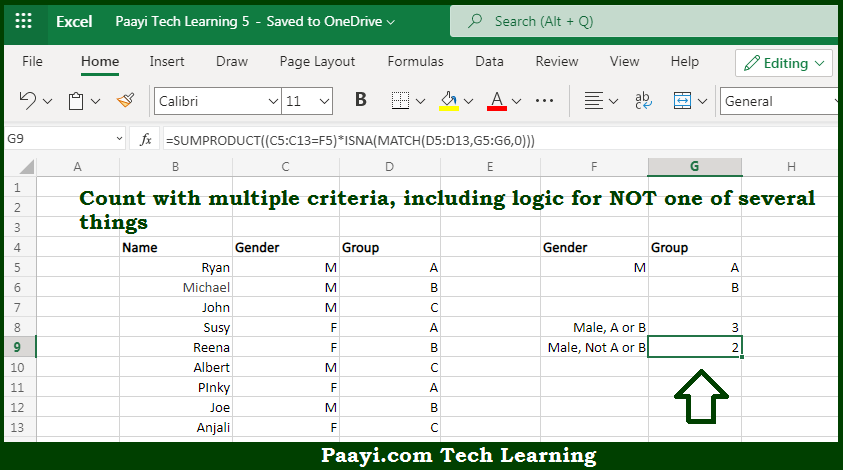# Learn How to Count Multiple Criteria With Not Logic in Microsoft Excel

Written by | 0 Comments | 515 Views

In this article, you will learn how to COUNT various things in Microsoft Excel using a single or combination of functions and its purpose in Microsoft Excel. You will also get to know how to Count Multiple Criteria With Not Logic & see the generic formula

Count Multiple Criteria With Not Logic in Microsoft Excel

The main purpose of this formula is to count with multiple criteria, including logic for NOT one of several things. Here we will learn how to count In this article, you will learn how to COUNT various things in Mircosoft Excel using a single or combination of functions and its purpose in Microsoft Excel. You will also get to know how to Count Multiple Criteria With Not Logic and see the generic formula. multiple criteria with NOT Logic in Microsoft Excel. That implies, with the help of a formula based on the SUMPRODUCT function with ISNA & MATCH you can able to count with multiple criteria, including logic for NOT one of several things. So, with the help of this formula, you can able to count multiple criteria with NOT Logic.

General Formula to Count Multiple Criteria With Not Logic

=SUMPRODUCT((rng1=crit1)*ISNA(MATCH(rng2,crit2,0)))

The Explanation for the Count Multiple Criteria With Not LogicSo we know that with the help of the given formula above you can able to count with multiple criteria, including logic for NOT one of several things. Here we will learn how to count multiple criteria with NOT Logic in Microsoft Excel. As we know that, The first expression inside of SUMPRODUCTS tests values in column C. The second expression inside of SUMPRODUCTS tests values in column D. The MATCH function is used to match every value in the named range "group" against values in G4:G5, "A" and "B" So, with the help of this formula, you can able to count with multiple criteria, including logic for NOT one of several things.